# does not dissociate Hydrochloric acid alkaline The pH of water is approximately 7, which means it...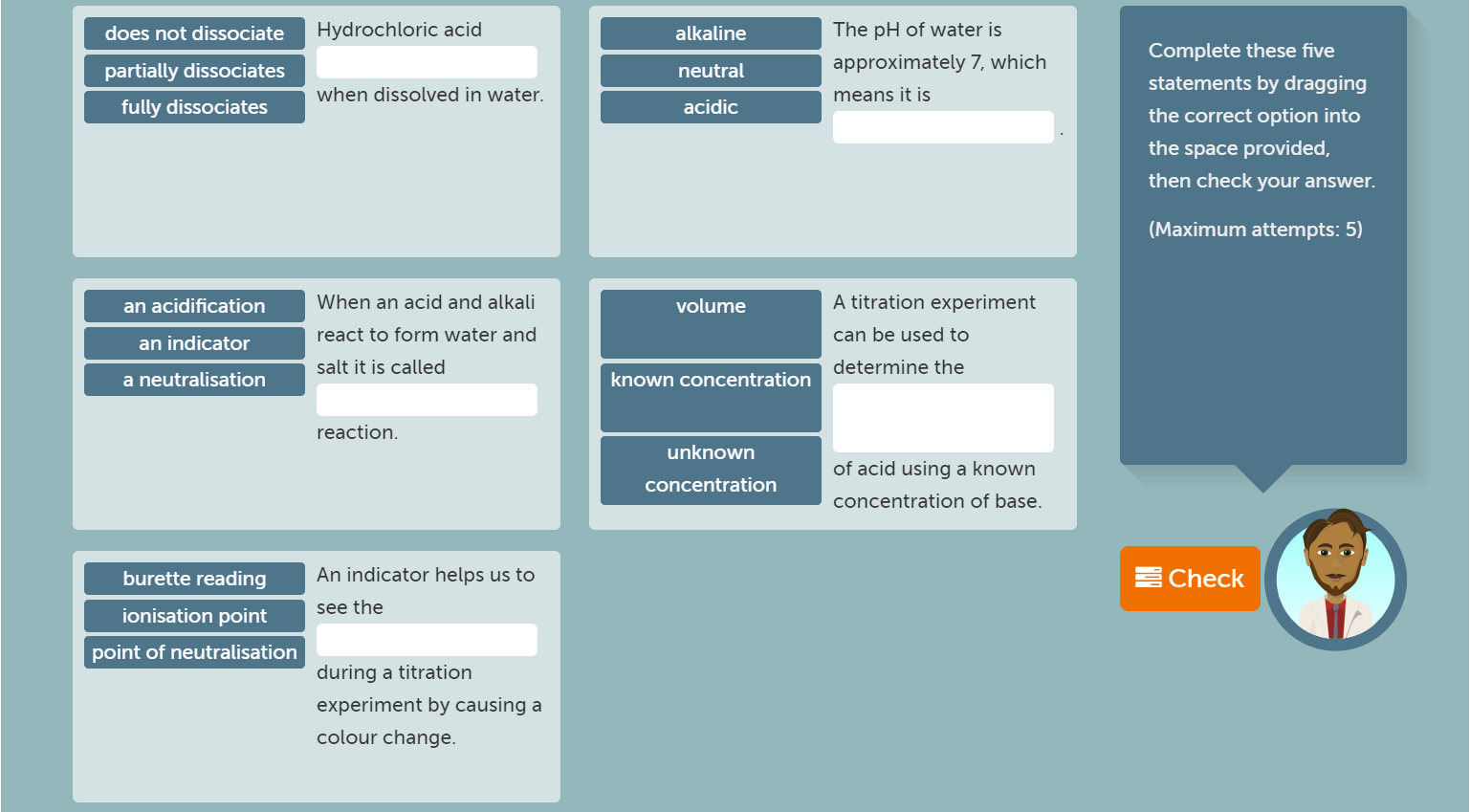does not dissociate Hydrochloric acid alkaline The pH of water is approximately 7, which means it is neutral partially dissociates fully dissociates when dissolved in water. acidic Complete these five statements by dragging the correct option into the space provided, then check your answer. (Maximum attempts: 5) an acidification volume an indicator a neutralisation When an acid and alkali react to form water and salt it is called A titration experiment can be used to determine the known concentration reaction. unknown concentration of acid using a known concentration of base. burette reading ionisation point point of neutralisation An indicator helps us to see the Check during a titration experiment by causing a colour change.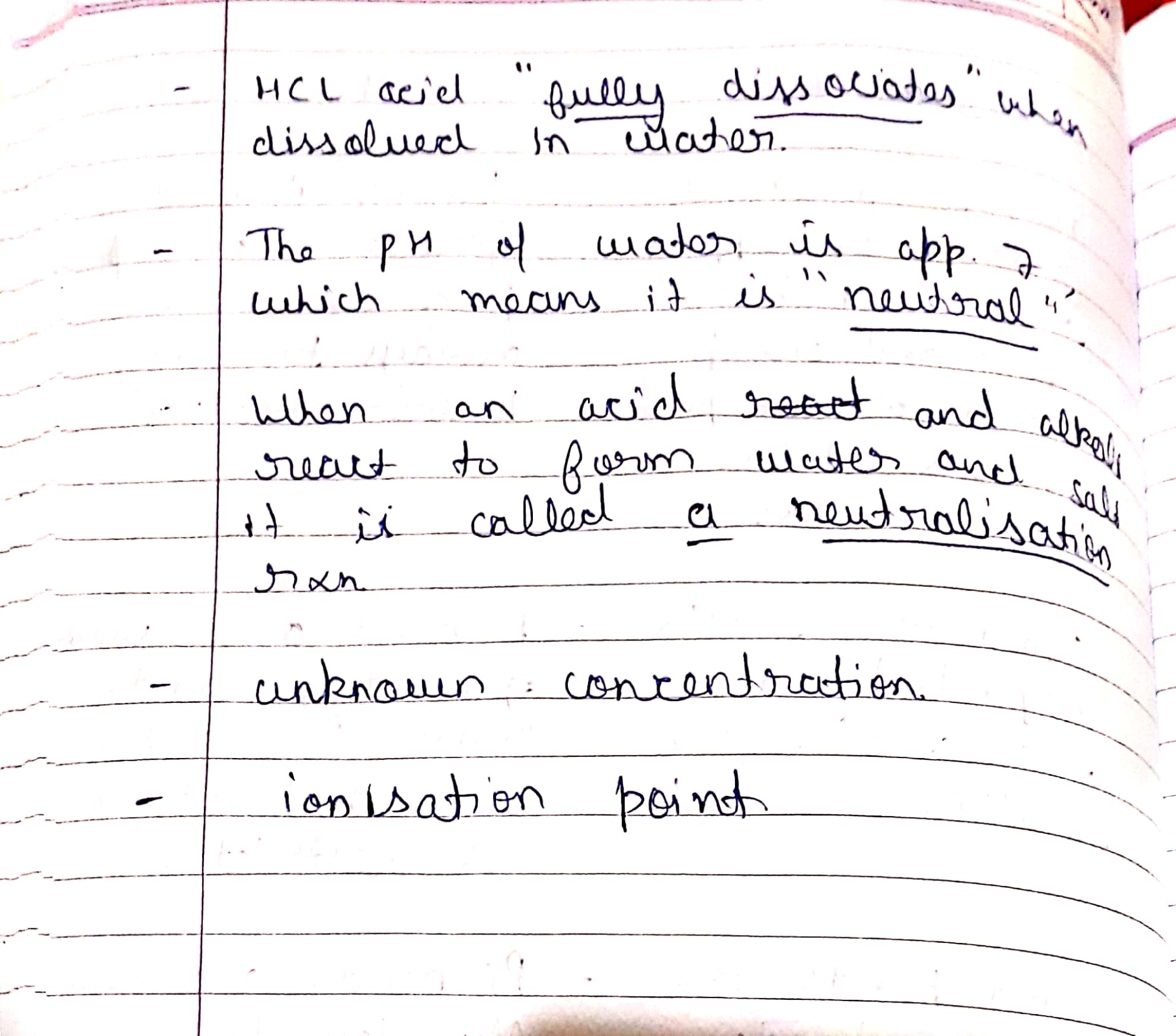#### Earn Coin

Coins can be redeemed for fabulous gifts.

Similar Homework Help Questions
• ### 1. Write the balanced equation for the reaction between calcium carbonate and hydrochloric acid in water,...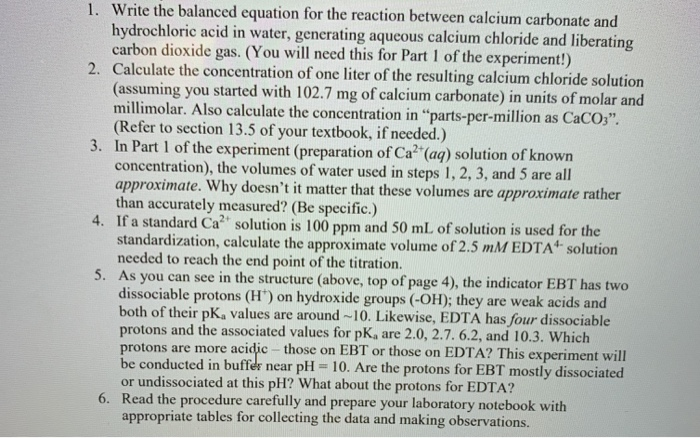1. Write the balanced equation for the reaction between calcium carbonate and hydrochloric acid in water, generating aqueous calcium chloride and liberating carbon dioxide gas. (You will need this for Part 1 of the experiment!) 2. Calculate the concentration of one liter of the resulting calcium chloride solution (assuming you started with 102.7 mg of calcium carbonate) in units of molar and millimolar. Also calculate the concentration in "parts-per-million as CaCO3". (Refer to section 13.5 of your textbook, if needed.)...

• ### Titration: Acids and Bases 2. How can you determine which acid is diprotic? 3. using the...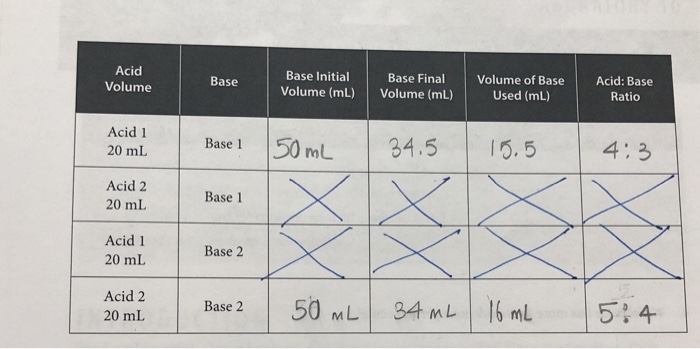Titration: Acids and Bases 2. How can you determine which acid is diprotic? 3. using the answers to questions one and two, which acid is diprotic? 4. Which base has more hydroxide ions per molecule? Acid Volume Base Base Initial Volume (mL) Base Final Volume (mL) Volume of Base Used (mL) Acid: Base Ratio Acid 1 20 mL Base 1 50 mL 34.5 15.5 4:3 Acid 2 20 mL Base 1 Acid 1 20 mL Base 2 Acid 2 20...

• ### Standardization of NaOH: Acid Base Titration Objective: In this lab, you will accurately determine the concentration...

Standardization of NaOH: Acid Base Titration Objective: In this lab, you will accurately determine the concentration of a solution of sodium hydroxide (NaOH) using a 0.500M potassium hydrogen phthalate (KHP) standard solution. Background: Acid–Base Titrations When an acid reacts with a base, a neutralization reaction occurs. The H+ ions from the acid and the HO– ions from the base combine to form water and are therefore neutralized. The other product of reaction is a salt. For example, hydrochloric acid reacts...

• ### It's a weak acid strong base titration Experiment 4: Identification of an unknown acid by titration...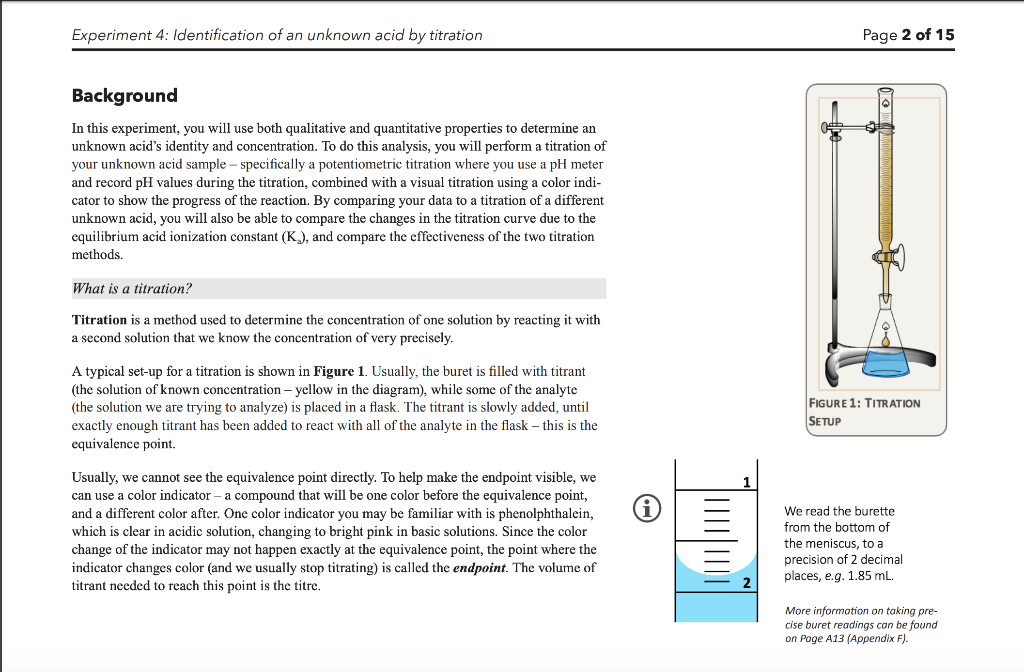It's a weak acid strong base titration Experiment 4: Identification of an unknown acid by titration Page 2 of 15 Background In this experiment, you will use both qualitative and quantitative properties to determine an unknown acid's identity and concentration. To do this analysis, you will perform a titration of your unknown acid sample-specifically a potentiometric titration where you use a pH meter and record pH values during the titration, combined with a visual titration using a color indi- cator...

• ### CHEM LAB hello can someone help me come up with a procedure for this lab it...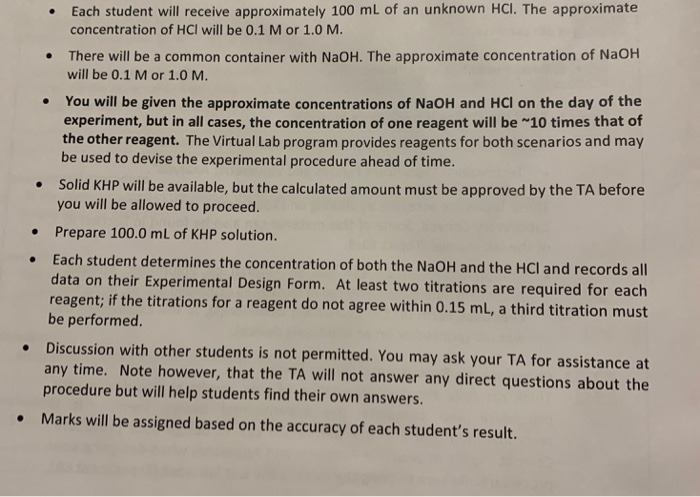CHEM LAB hello can someone help me come up with a procedure for this lab it is an standarization of acid and base solution • Each student will receive approximately 100 mL of an unknown HCl. The approximate concentration of HCl will be 0.1 M or 1.0 M. There will be a common container with NaOH. The approximate concentration of NaOH will be 0.1 M or 1.0 M. You will be given the approximate concentrations of NaOH and HCl on...

• ### please use the information provided above to answer the questions below (the 2 worksheet) _35%. 1:59...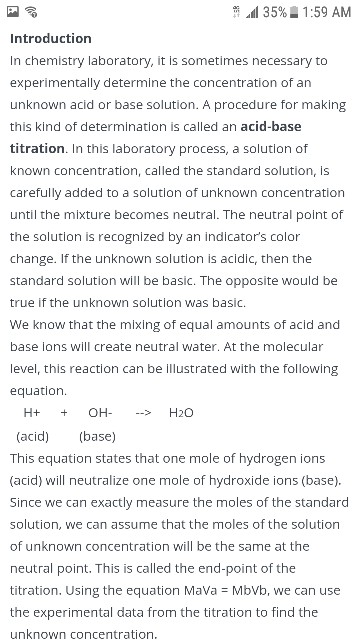please use the information provided above to answer the questions below (the 2 worksheet) _35%. 1:59 AM Introduction In chemistry laboratory, it is sometimes necessary to experimentally determine the concentration of an unknown acid or base solution. A procedure for making this kind of determination is called an acid-base titration. In this laboratory process, a solution of known concentration, called the standard solution, is carefully added to a solution of unknown concentration until the mixture becomes neutral. The neutral point...

• ### please use the information provided to fill out the first sheet (the data) Data Sheet: 1....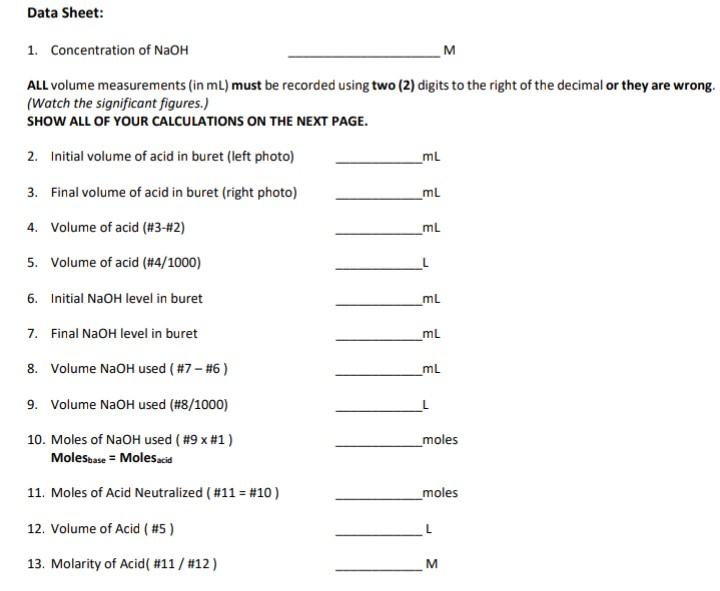please use the information provided to fill out the first sheet (the data) Data Sheet: 1. Concentration of NaOH ALL volume measurements (in mL) must be recorded using two (2) digits to the right of the decimal or they are wrong. (Watch the significant figures.) SHOW ALL OF YOUR CALCULATIONS ON THE NEXT PAGE. 2. Initial volume of acid in buret (left photo) 3. Final volume of acid in buret (right photo) 4. Volume of acid (#3 #2) 5. Volume...

• ### 2) What chemical reaction does the titrant contribute to this experiment? CHEM 1A Experiment #8: Measuring the Vit...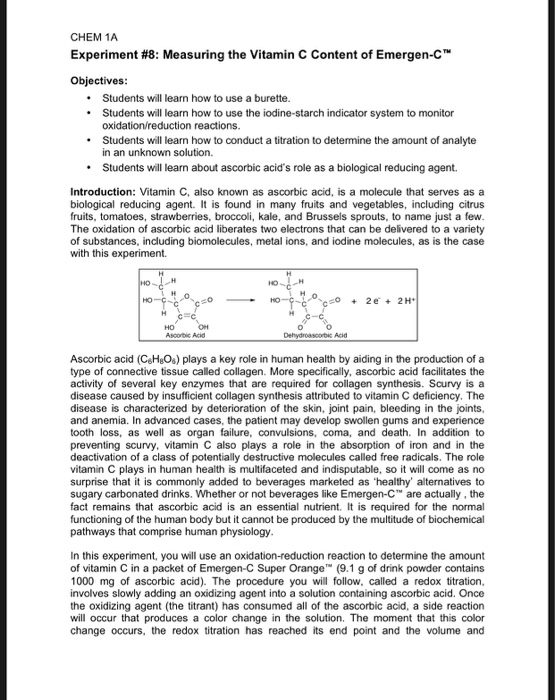2) What chemical reaction does the titrant contribute to this experiment? CHEM 1A Experiment #8: Measuring the Vitamin C Content of Emergen-C™ Objectives: Students will learn how to use a burette. Students will learn how to use the iodine starch indicator system to monitor oxidation/reduction reactions. • Students will learn how to conduct a titration to determine the amount of analyte in an unknown solution. • Students will learn about ascorbic acid's role as a biological reducing agent. Introduction: Vitamin...

• ### just need help with questions 3. a & b always rate!!! Introduction Titration is a common...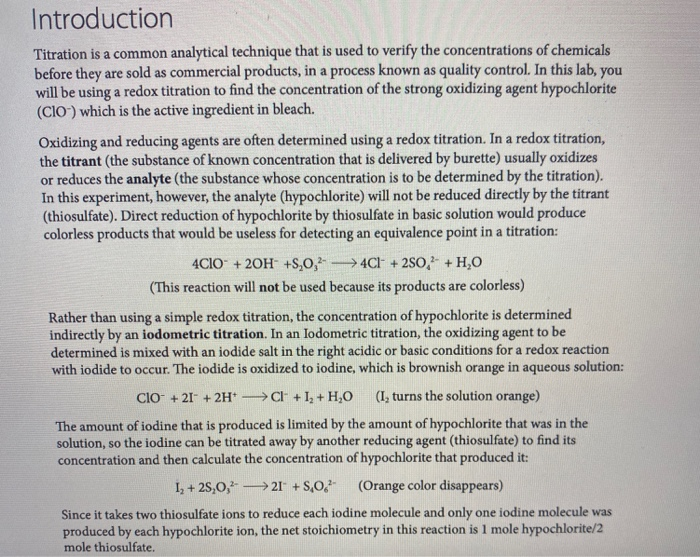just need help with questions 3. a & b always rate!!! Introduction Titration is a common analytical technique that is used to verify the concentrations of chemicals before they are sold as commercial products, in a process known as quality control. In this lab, you will be using a redox titration to find the concentration of the strong oxidizing agent hypochlorite (CIO) which is the active ingredient in bleach. Oxidizing and reducing agents are often determined using a redox titration....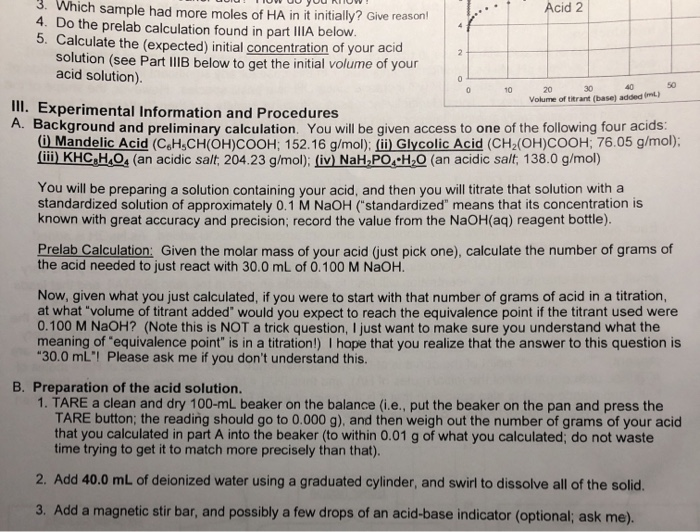please help me with 3,4,5 Thank you! 3. Which sample had more moles of HA in it initially? Give reas on ! 4. Do the prelab calculation found in part IIIA below 5. Calculate the (expected) initial concentration of your acid solution (see Part IIIB below to get the initial volume of your acid solution) Acid 2 0 50 40 30 0 10 20 Volume of titrant (base) added (mL) III. Experimental Information and Procedures A.Background and preliminary calculation. You...